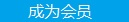您可以捐助，支持我们的公益事业。 1元 10元 50元 认证码：必填求知 文章 文库 Lib 视频 Code iProcess 课程 角色 咨询 工具 火云堂 讲座吧 建模者要资料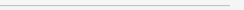订阅捐助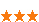1028 次浏览     评价： 好 中 差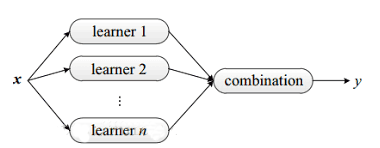bagging

bagging的特点

1.训练一个bagging集成与直接使用基学习算法训练一个学习器的复杂度同阶

2.与标准的adboost只适用于二分类任务不同的是，bagging能不经修改地用于多分类、回归等任务

3.由于自助采样过程的性质，包外样本可以用作包外估计，可用来辅助剪枝，减小过拟合风险

4.从偏差-方差角度看，bagging主要关注降低方差，因此它在不剪枝决策树、神经网络等易受样本扰动的学习器上效用更明显。

boosting

boosting是一种与bagging很类似的技术。不论是boosting还是bagging，所使用的分类器的类型都是一致的。但是bagging是个体学习器间不存在强依赖关系、可同时生成的并行化方法；boosting是个体学习器间存在强依赖关系、必须串行生成的序列化方法。

boosting不同的分类器是通过串行训练而获得的，每个新分类器都根据已训练出的分类器性能来进行训练。boosting是通过集中关注被已有分类器错分的那些数据来获得新的分类器。

boosting族算法最具代表性的是AdaBoost。关于AdaBoost我前面有篇博客有分享：利用AdaBoost元算法提高分类性能。这里我将结合那篇博客的内容深入分析AdaBoost的原理与实现。

AdaBoost算法有很多的推导方式，比较容易理解的是基于“加性模型”，即基学习器的线性组合：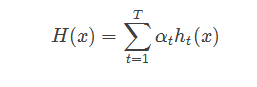AdaBoost是adaptive boosting的缩写，其运行过程如下：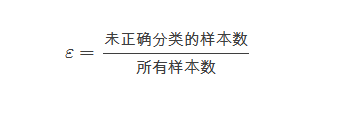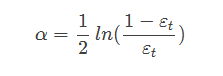AdaBoost算法的流程如下图所示：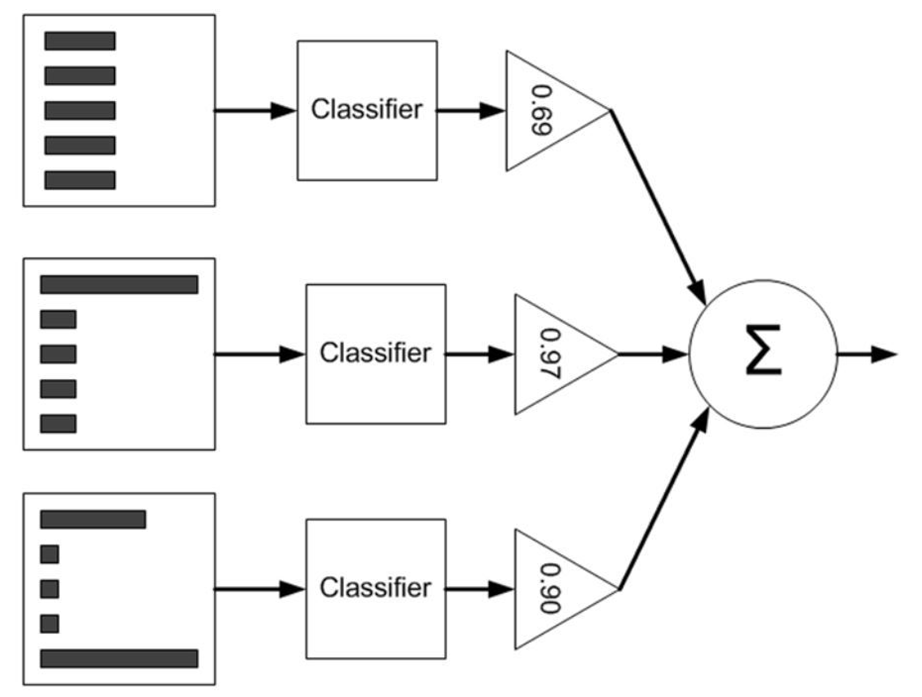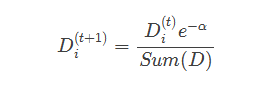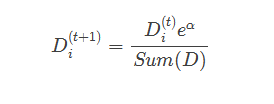AdaBoost的实现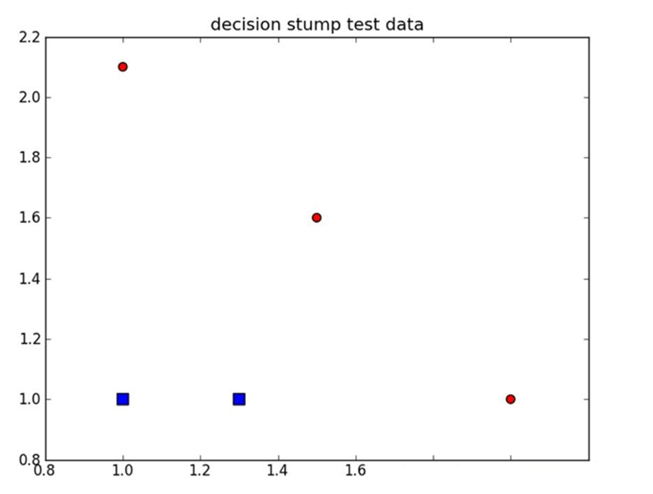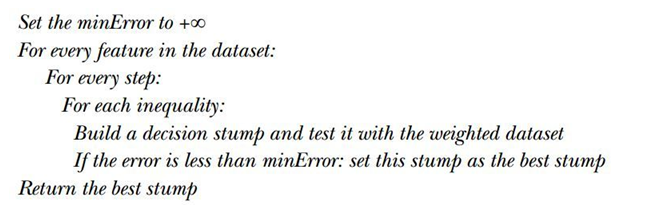def stumpClassify(dataMatrix,dimen,threshVal, threshIneq):#just classify the data retArray = ones((shape(dataMatrix),1)) if threshIneq == 'lt': retArray[dataMatrix[:,dimen] <= threshVal] = -1.0 else: retArray[dataMatrix[:,dimen] > threshVal] = -1.0 return retArray def buildStump(dataArr,classLabels,D): dataMatrix = mat(dataArr); labelMat = mat (classLabels).T m,n = shape(dataMatrix) numSteps = 10.0; bestStump = {}; bestClasEst = mat(zeros((m,1))) minError = inf #init error sum, to +infinity for i in range(n):#loop over all dimensions rangeMin = dataMatrix[:,i].min(); rangeMax = dataMatrix[:,i].max(); stepSize = (rangeMax-rangeMin)/numSteps for j in range(-1,int(numSteps)+1):#loop over all range in current dimension for inequal in ['lt', 'gt']: #go over less than and greater than threshVal = (rangeMin + float(j) * stepSize) predictedVals = stumpClassify(dataMatrix,i, threshVal,inequal)#call stump classify with i, j, lessThan errArr = mat(ones((m,1))) errArr[predictedVals == labelMat] = 0 weightedError = D.T*errArr #calc total error multiplied by D #print "split: dim %d, thresh %.2f, thresh ineqal: %s, the weighted error is %.3f" % (i, threshVal, inequal, weightedError) if weightedError < minError: minError = weightedError bestClasEst = predictedVals.copy() bestStump['dim'] = i bestStump['thresh'] = threshVal bestStump['ineq'] = inequal return bestStump,minError,bestClasEst

AdaBoost训练

AdaBoost训练的伪代码构造如下：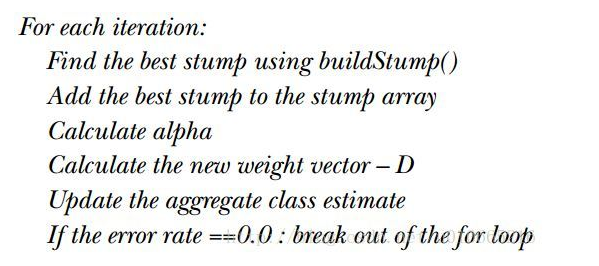def adaBoostTrainDS(dataArr,classLabels,numIt=40): weakClassArr = [] m = shape(dataArr) D = mat(ones((m,1))/m) #init D to all equal aggClassEst = mat(zeros((m,1))) for i in range(numIt): bestStump,error,classEst = buildStump(dataArr,classLabels,D)#build Stump #print "D:",D.T alpha = float(0.5*log((1.0-error)/max(error,1e-16)))#calc alpha, throw in max(error,eps) to account for error=0 bestStump['alpha'] = alpha weakClassArr.append(bestStump) #store Stump Params in Array #print "classEst: ",classEst.T expon = multiply(-1*alpha*mat(classLabels).T,classEst) #exponent for D calc, getting messy D = multiply(D,exp(expon)) #Calc New D for next iteration D = D/D.sum() #calc training error of all classifiers, if this is 0 quit for loop early (use break) aggClassEst += alpha*classEst #print "aggClassEst: ",aggClassEst.T aggErrors = multiply(sign(aggClassEst) != mat(classLabels).T,ones((m,1))) errorRate = aggErrors.sum()/m print "total error: ",errorRate if errorRate == 0.0: break return weakClassArr,aggClassEst

AdaBoost测试

 def adaClassify(datToClass,classifierArr): dataMatrix = mat(datToClass)#do stuff similar to last aggClassEst in adaBoostTrainDS m = shape(dataMatrix) aggClassEst = mat(zeros((m,1))) for i in range(len(classifierArr)): classEst = stumpClassify(dataMatrix,classifierArr[i]['dim'],\ classifierArr[i]['thresh'],\ classifierArr[i]['ineq'])#call stump classify aggClassEst += classifierArr[i]['alpha']*classEst print aggClassEst return sign(aggClassEst)

Adaboost应用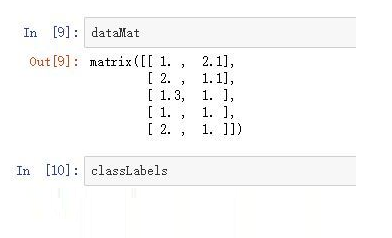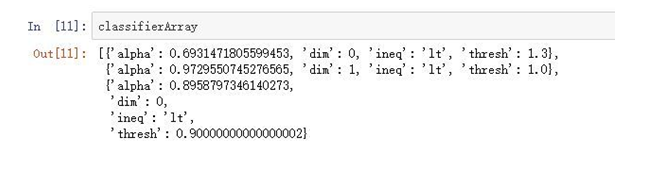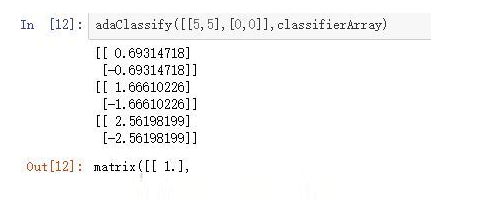1028 次浏览  评价: 好 中 差订阅捐助
 相关文章 为什么要做持续部署？ 剖析“持续交付”：五个核心实践 集成与构建指南 持续集成工具的选择-装载
 相关文档 持续集成介绍 使用Hudson持续集成 持续集成之-依赖管理 IPD集成产品开发管理
 相关课程 配置管理、日构建与持续集成 软件架构设计方法、案例与实践 单元测试、重构及持续集成 基于Android的单元、性能测试每天2个文档/视频 扫描微信二维码订阅
 订阅技术月刊 获得每月300个技术资源